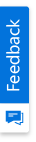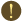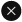We use cookies to give you the best experience on our website. If you continue to browse, then you agree to our privacy policy and cookie policy.Unfortunately, activation email could not send to your email. Please try again.# How can we avoid the 'Unexpected token' exception while using formula in XlsIO?

Platform: WinForms |
Control: XlsIO

Syncfusion Excel (XlsIO) library is a .NET Excel library used to create, read, and edit Excel documents. Also, converts Excel documents to PDF files. Using this library, you can use formulas within and across worksheets.

While working with formulas, sometimes you may encounter ‘Unexpected token’ exception. This article explains how we can avoid unexpected token exception while using formulas in XlsIO.

## What is unexpected token exception?

Unexpected token exception occurs when some arguments or tokens are misplaced or misused in a formula. In Syncfusion Excel (XlsIO) library, while parsing the formula, it is split into tokens. When a token is not valid, unexpected token exception will be thrown.

Argument separator in a formula can be a reason for the exception. Unexpected token exception occurs if different regional setting is used other than English and comma is used in formula. You must set separator to workbook before assigning formula depending upon your current UI culture.

For example, consider that we need to add two numbers using formula as below.

C#

```//Data to be used in formula
worksheet["B1"].Number = 10;
worksheet["B2"].Number = 20;

//Formula to add two numbers
worksheet["B3"].Formula = "=SUM(B1,B2)";
```

VB.NET

```‘Data to be used in formula
worksheet("B1").Number = 10
worksheet("B2").Number = 20

'Formula to add two numbers
worksheet("B3").Formula = "=SUM(B1,B2)"
```

In this case, we can avoid this exception by setting arguments separator as ‘;’ before using the formula.

C#

```//Set separators for formula parsing
workbook.SetSeparators(';', ',');
```

VB.NET

```'Set separators for formula parsing
workbook.SetSeparators(";", ",")
```

## Steps to avoid the exception while using formulas in XlsIO, programmatically:

Step 1: Create a new C# console application project.Create a new C# console application

Step 2: Install the Syncfusion.XlsIO.WinForms NuGet package as reference to your .NET Framework application from NuGet.org.Install NuGet package

Step 3: Include the following namespace in the Program.cs file.

C#

```using Syncfusion.XlsIO;
```

VB.NET

```Imports Syncfusion.XlsIO
```

Step 4: The following C#, VB.NET code shows how to avoid unexpected token exception while using formulas in XlsIO

C#

```//Instantiate the spreadsheet creation engine
using (ExcelEngine excelEngine = new ExcelEngine())
{
IApplication application = excelEngine.Excel;
IWorkbook workbook = application.Workbooks.Create(1);
IWorksheet worksheet = workbook.Worksheets;

//Set separators for formula parsing
workbook.SetSeparators(';', ',');

worksheet["A1"].Text = "Value 1";
worksheet["A2"].Text = "Value 2";
worksheet["A3"].Text = "Sum";

worksheet["B1"].Number = 10;
worksheet["B2"].Number = 20;

//Formula to add two numbers
worksheet["B3"].Formula = "=SUM(B1;B2)";

//Save and close the workbook
worksheet.UsedRange.AutofitColumns();
workbook.SaveAs("Output.xlsx");
}
```

VB.NET

```'Instantiate the spreadsheet creation engine
Using excelEngine As ExcelEngine = New ExcelEngine()
Dim application As IApplication = excelEngine.Excel
Dim workbook As IWorkbook = application.Workbooks.Create(1)
Dim worksheet As IWorksheet = workbook.Worksheets(0)

'Set separators for formula parsing
workbook.SetSeparators(";", ",")

worksheet("A1").Text = "Value 1"
worksheet("A2").Text = "Value 2"
worksheet("A3").Text = "Sum"

worksheet("B1").Number = 10
worksheet("B2").Number = 20

'Formula to add two numbers
worksheet("B3").Formula = "=SUM(B1;B2)"

'Save and close the workbook
worksheet.UsedRange.AutofitColumns()
workbook.SaveAs("Output.xlsx")
End Using
```

A complete working example of how to avoid unexpected token exception while using formulas in XlsIO can be downloaded from Avoid Unexpected Token Exception.zip.

By executing the program, you will get the output Excel file as shown below:Output Excel document

Tip:

If you face this exception in other similar cases, initially you should check whether all the tokens in the formula are used correctly. Also, separator in formula should match the current UI culture. If you are not sure about the separator to be used, set separators on your own before using the formula.

To know more about formulas in Syncfusion Excel (XlsIO) library, please refer the documentation.

Refer here to explore the rich set of Syncfusion Excel (XlsIO) library features.

I face different exceptions after upgraded XlsIO to the version 6.2 and later. Is there a solution available?

How to avoid generic error occurred in GDI+ while saving EMF image in Azure?

Why the “type initializer exception” thrown while running the application in production server?

Why the “Formula string can’t be empty” exception was thrown?

Note:

Starting with v16.2.0.x, if you reference Syncfusion assemblies from trial setup or from the NuGet feed, include a license key in your projects. Refer the link to learn about generating and registering Syncfusion license key in your application to use the components without trail message.

2X faster development

The ultimate WinForms UI toolkit to boost your development speed.You are using an outdated version of Internet Explorer that may not display all features of this and other websites. Upgrade to Internet Explorer 8 or newer for a better experience.